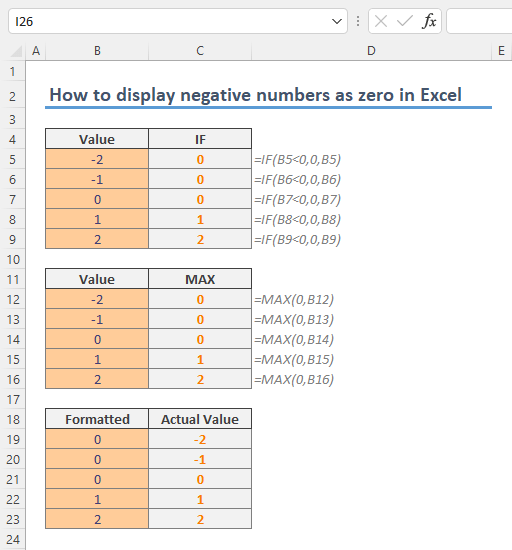## How to generate Descriptive Statistics in Excel

Descriptive statistics are summarizations of your dataset. This summary includes mean, median, mode, standard deviation, variance, minimum and maximum variables, kurtosis, and skewness. Excel can generate all these measures for a selected range in a proper table. In...## How to use subtotal feature in Excel

A subtotal is the aggregation of a data set, typically showing the totals of a certain section in that data set. Excel gives you tools to calculate subtotals in different ways. In this article, we are going to show how to use the subtotal feature in Excel. Download...## How to calculate as-the-crow-flies distance between two cities in Excel

You might think "as the crow flies" distance is nothing more than a simple hypotenuse calculation back from geometry class. Unfortunately, not. You should work with either decimal degrees or degree-minute-second (DMS) notation due to the curvy structure of Earth. This...## A Use Case of Excel's LAMBDA Function in SpreadsheetWeb: Splitting Text by delimiter with LAMBDA Function

SpreadsheetWeb Hub supports Excel's LAMBDA function. The recently released LAMBDA function allows Excel users to build custom, reusable functions without VBA. There are so many use cases of the LAMBDA function. In this article, we will create a LAMBDA Function to...## A Use Case of Excel's LAMBDA Function in SpreadsheetWeb: Calculating Distance by Latitude and Longitude

SpreadsheetWeb Hub supports Excel's LAMBDA function. Recently released LAMBDA function allows Excel users to build custom, reusable functions without VBA. There are so many use cases of LAMBDA function. In this article, we will build a function to calculate distance...## How to display negative numbers as zero in Excel

In this guide, we’re going to show you How to display negative numbers as zero in Excel in two ways. Download-Workbook Formula approach to display negative numbers as zero The first method in our list is the formula way. An obvious way is to use the IF function which...